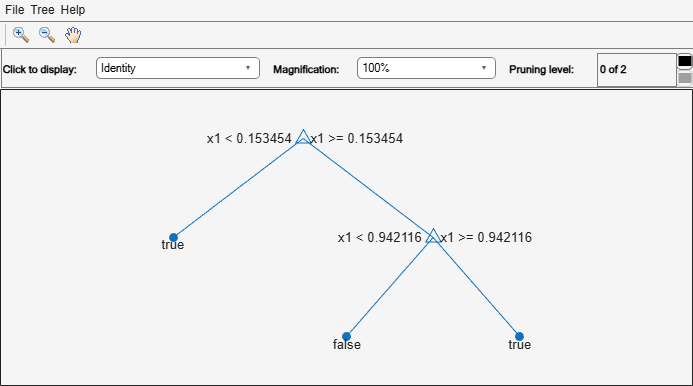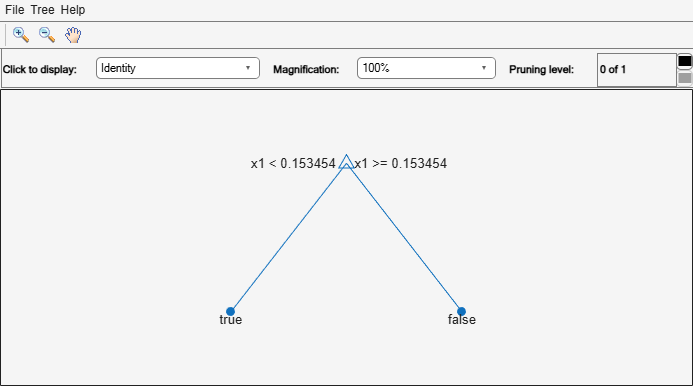# resubPredict

Class: ClassificationTree

Predict resubstitution labels of classification tree

## Syntax

```label = resubPredict(tree) [label,posterior] = resubPredict(tree) [label,posterior,node] = resubPredict(tree) [label,posterior,node,cnum] = resubPredict(tree) [label,...] = resubPredict(tree,Name,Value) ```

## Description

`label = resubPredict(tree)` returns the labels `tree` predicts for the data `tree.X`. `label` is the predictions of `tree` on the data that `fitctree` used to create `tree`.

```[label,posterior] = resubPredict(tree)``` returns the posterior class probabilities for the predictions.

```[label,posterior,node] = resubPredict(tree)``` returns the node numbers of `tree` for the resubstituted data.

```[label,posterior,node,cnum] = resubPredict(tree)``` returns the predicted class numbers for the predictions.

`[label,...] = resubPredict(tree,Name,Value)` returns resubstitution predictions with additional options specified by one or more `Name,Value` pair arguments.

## Input Arguments

expand all

 `tree` A classification tree constructed by `fitctree`.

### Name-Value Arguments

Specify optional pairs of arguments as `Name1=Value1,...,NameN=ValueN`, where `Name` is the argument name and `Value` is the corresponding value. Name-value arguments must appear after other arguments, but the order of the pairs does not matter.

Before R2021a, use commas to separate each name and value, and enclose `Name` in quotes.

Pruning level, specified as the comma-separated pair consisting of `'Subtrees'` and a vector of nonnegative integers in ascending order or `'all'`.

If you specify a vector, then all elements must be at least `0` and at most `max(tree.PruneList)`. `0` indicates the full, unpruned tree and `max(tree.PruneList)` indicates the completely pruned tree (i.e., just the root node).

If you specify `'all'`, then `resubPredict` operates on all subtrees (i.e., the entire pruning sequence). This specification is equivalent to using `0:max(tree.PruneList)`.

`resubPredict` prunes `tree` to each level indicated in `Subtrees`, and then estimates the corresponding output arguments. The size of `Subtrees` determines the size of some output arguments.

To invoke `Subtrees`, the properties `PruneList` and `PruneAlpha` of `tree` must be nonempty. In other words, grow `tree` by setting `'Prune','on'`, or by pruning `tree` using `prune`.

Example: `'Subtrees','all'`

Data Types: `single` | `double` | `char` | `string`

## Output Arguments

 `label` The response `tree` predicts for the training data. `label` is the same data type as the training response data `tree.Y`. If the `Subtrees` name-value argument contains `m`>`1` entries, `label` has `m` columns, each of which represents the predictions of the corresponding subtree. Otherwise, `label` is a vector. `posterior` Matrix or array of posterior probabilities for classes `tree` predicts. If the `Subtrees` name-value argument is a scalar or is missing, `posterior` is an `n`-by-`k` matrix, where `n` is the number of rows in the training data `tree.X`, and `k` is the number of classes. If `Subtrees` contains `m`>`1` entries, `posterior` is an `n`-by-`k`-by-`m` array, where the matrix for each `m` gives posterior probabilities for the corresponding subtree. `node` The node numbers of `tree` where each data row resolves. If the `Subtrees` name-value argument is a scalar or is missing, `node` is a numeric column vector with `n` rows, the same number of rows as `tree.X`. If `Subtrees` contains `m`>`1` entries, `node` is a `n`-by-`m` matrix. Each column represents the node predictions of the corresponding subtree. `cnum` The class numbers that `tree` predicts for the resubstituted data. If the `Subtrees` name-value argument is a scalar or is missing, `cnum` is a numeric column vector with `n` rows, the same number of rows as `tree.X`. If `Subtrees` contains `m`>`1` entries, `cnum` is a `n`-by-`m` matrix. Each column represents the class predictions of the corresponding subtree.

## Examples

expand all

Find the total number of misclassifications of the Fisher iris data for a classification tree.

```load fisheriris tree = fitctree(meas,species); Ypredict = resubPredict(tree); % The predictions Ysame = strcmp(Ypredict,species); % True when == sum(~Ysame) % How many are different?```
```ans = 3 ```

Load Fisher's iris data set. Partition the data into training (50%)

`load fisheriris`

Grow a classification tree using the all petal measurements.

```Mdl = fitctree(meas(:,3:4),species); n = size(meas,1); % Sample size K = numel(Mdl.ClassNames); % Number of classes```

View the classification tree.

`view(Mdl,'Mode','graph');`The classification tree has four pruning levels. Level 0 is the full, unpruned tree (as displayed). Level 4 is just the root node (i.e., no splits).

Estimate the posterior probabilities for each class using the subtrees pruned to levels 1 and 3.

`[~,Posterior] = resubPredict(Mdl,'SubTrees',[1 3]);`

`Posterior` is an `n`-by- `K`-by- 2 array of posterior probabilities. Rows of `Posterior` correspond to observations, columns correspond to the classes with order `Mdl.ClassNames`, and pages correspond to pruning level.

Display the class posterior probabilities for iris 125 using each subtree.

`Posterior(125,:,:)`
```ans = ans(:,:,1) = 0 0.0217 0.9783 ans(:,:,2) = 0 0.5000 0.5000 ```

The decision stump (page 2 of `Posterior`) has trouble predicting whether iris 125 is versicolor or virginica.

Classify a predictor `X` as true when `X < 0.15` or `X > 0.95`, and as false otherwise.

Generate 100 uniformly distributed random numbers between 0 and 1, and classify them using a tree model.

```rng("default") % For reproducibility X = rand(100,1); Y = (abs(X - 0.55) > 0.4); tree = fitctree(X,Y); view(tree,"Mode","graph")```Prune the tree.

```tree1 = prune(tree,"Level",1); view(tree1,"Mode","graph")```The pruned tree correctly classifies observations that are less than 0.15 as `true`. It also correctly classifies observations from 0.15 to 0.95 as `false`. However, it incorrectly classifies observations that are greater than 0.95 as `false`. Therefore, the score for observations that are greater than 0.15 should be about 0.05/0.85=0.06 for `true`, and about 0.8/0.85=0.94 for `false`.

Compute the prediction scores (posterior probabilities) for the first 10 rows of `X`.

```[~,score] = resubPredict(tree1); [score(1:10,:) X(1:10)]```
```ans = 10×3 0.9059 0.0941 0.8147 0.9059 0.0941 0.9058 0 1.0000 0.1270 0.9059 0.0941 0.9134 0.9059 0.0941 0.6324 0 1.0000 0.0975 0.9059 0.0941 0.2785 0.9059 0.0941 0.5469 0.9059 0.0941 0.9575 0.9059 0.0941 0.9649 ```

Indeed, every value of `X` (the right-most column) that is less than 0.15 has associated scores (the left and center columns) of 0 and 1, while the other values of `X` have associated scores of approximately 0.91 and 0.09. The difference (score of 0.09 instead of the expected 0.06) is due to a statistical fluctuation: there are 8 observations in `X` in the range (0.95,1) instead of the expected 5 observations.

`sum(X > 0.95)`
```ans = 8 ```